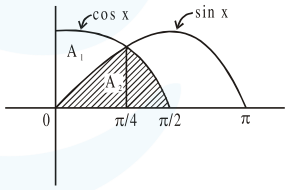# Solve the Following Questions

Question:

Let $A_{1}$ be the area of the region bounded by the curves $y=\sin x, y=\cos x$ and $y$-axis in the first quadrant. Also, let $\mathrm{A}_{2}$ be the area of the region bounded by the curves $y=\sin x$,

$y=\cos x, x$-axis and $x=\frac{\pi}{2}$ in the first quadrant.

Then,

1. $\mathrm{A}_{1}: \mathrm{A}_{2}=1: \sqrt{2}$ and $\mathrm{A}_{1}+\mathrm{A}_{2}=1$

2. $\mathrm{A}_{1}=\mathrm{A}_{2}$ and $\mathrm{A}_{1}+\mathrm{A}_{2}=\sqrt{2}$

3. $2 \mathrm{~A}_{1}=\mathrm{A}_{2}$ and $\mathrm{A}_{1}+\mathrm{A}_{2}=1+\sqrt{2}$

4. $\mathrm{A}_{1}: \mathrm{A}_{2}=1: 2$ and $\mathrm{A}_{1}+\mathrm{A}_{2}=1$

Correct Option: 1

Solution:$A_{1}=\int_{0}^{\pi / 4}(\cos x-\sin x) d x$

$A_{1}=(\sin x+\cos x)_{0}^{\pi / 4}=\sqrt{2}-1$

$\mathrm{A}_{2}=\int_{0}^{\pi / 4} \sin \mathrm{x} \mathrm{dx}+\int_{\pi / 4}^{\pi / 2} \cos \mathrm{x} \mathrm{dx}$

$=(-\cos x)_{0}^{\pi / 4}+(\sin x)_{\pi / 4}^{\pi / 2}$

$\mathrm{A}_{2}=\sqrt{2}(\sqrt{2}-1)$

$\mathrm{A}_{1}: \mathrm{A}_{2}=1: \sqrt{2}, \mathrm{~A}_{1}+\mathrm{A}_{2}=1$